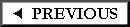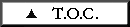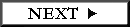## ITEMIZATION OF LOADS

Before you can begin to determine the size of your framing members you need to know the total of the loads to be supported. The following are typical summaries (often called "Loading Lists"). These are examples only - your materials will probably be different than those listed.

For A Roof...

1. Wood shingles = 3.0 psf
2. 1 x 4 nailers at 8" o.c. = (1/2)(2 x 4 at 12" o.c.)(1.5) = 1.05 psf
3. 3 1/2" of batt insulation = (3.5)(0.30) = 1.05 psf
4. 2 x 4 rafters at 16" o.c. = 1.1 psf

Total Dead Loads = 3.0 + 1.05 + 1.05 + 1.1 = 6.2 psf

Live Load = 20 psf; may be less depending on roof slope.

For A Roof Which Also Supports A Ceiling...

1. All of the above = 6.2 psf
2. 5/8" gypsum board = 2.8 psf
3. Lights/Misc. = 1.0 psf

Total Dead Loads = 6.2 + 2.8 + 1.0 = 10.0 psf

Live Load = 20 psf; may be less depending on roof slope.

For The Bottom Floor Of A Single Story Residence, No Basement...

1. Hardwood flooring = 4.0 psf
2. 3/4" thick plywood subfloor = 2.3 psf
3. 2 x 8 at 16" o.c. = 2.2 psf
4. 6" Glass wool = (6)(0.30) = 1.8 psf

Total Dead Loads = 4.0 + 2.3 + 2.2 + 1.8 = 10.3 psf

Live Load = 40 psf for residential occupancies.

For A Floor Which Also Supports A Ceiling Below...

1. All of the above = 10.3 psf
2. Subtract the insulation = -1.8 psf
3. Add 5/8" gypsum board = +2.8 psf
4. Lights/Misc. = 1.0 psf

Total Dead Loads = 10.3 - 1.8 + 2.8 + 1.0 = 12.3 psf

Live Load = 40 psf for residential occupancies.